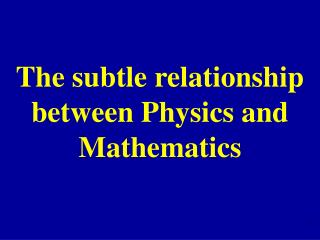# The subtle relationship between Physics and Mathematics - PowerPoint PPT PresentationDownload PresentationThe subtle relationship between Physics and Mathematics

The subtle relationship between Physics and MathematicsDownload Presentation## The subtle relationship between Physics and Mathematics

- - - - - - - - - - - - - - - - - - - - - - - - - - - E N D - - - - - - - - - - - - - - - - - - - - - - - - - - -
##### Presentation Transcript

1. The subtle relationship between Physics and Mathematics

2. I. • Physics of a neutron. • After 1926, the mathema-tics of QM shows that a Fermion rotated 360° does not come back to itself. It acquires a phase of -1.

3. Werner et al. PRL 35(1975)1053

4. II. Dirac’s Game

5. (1)After a rotation of 720°, could the strings be disentangled without moving the block? • (2) After a rotation of 360°, could the strings be disentangled without moving the block?

6. The answers to (1) and (2), (yes or no) cannot depend on the original positions of the strings.

7. 360° 720°

8. 360° 720°

9. 360° 720°

10. 360° 720°

11. Algebraic representations of braids (and knots).

12. 360° 720°

13. AA-1 = I A-1A = I

14. I (360°) A2 (720°)A4

15. (1) Is A4 = I ? • (2) Is A2 = I ?

16. A‧A-1 = I B‧B-1 = I

17. ABA BAB ABA = BAB Artin

18. ABBA = I

19. ABBA = IB-1A-1(ABBA)AB = B-1A-1IAB = IBAAB = I

20. ABA = BABABA • ABA = BAB • BAB A2 = B2ABBA = I → A4 = IHence answer to (1): Yes

21. The algebra of the last 3 slides shows how to do the disentangling.

22. A = B = i • A-1= B-1= -i • satisfy all 3 rules: • AA-1 = A-1A = BB-1 = B-1B = I • ABBA = I • BA = BAB

23. But A2 = -1 ≠ I • Hence answer to (2): No

24. III. • Mathematics of Knots

25. Planar projections of prime knots and links with six or fewer crossings.

26. Knots are related to Braids

27. Fundamental Problem of Knot Theory: How to classify all knots

28. Alexander Polynomial 1 1 + z2 1 + 3z2 + z4

29. Two knots with different Alexander Polynomials are inequivalent.

30. But Alexander Polynomial is not discriminating enough.

31. Both knots have Alexander Polynomial = 1 (from C. Adams: The Knot Book)

32. Jones Polynomial (1987) Homfly Polynomial Kauffman Polynomial etc.

33. Statistical Mechanics (Many Body Problem) 1967: Yang Baxter Equation

34. ABA = BAB • (12)(23)(12)=(23)(12)(23) • A(u)B(u+v)A(v) = B(v)A(u+v)B(u)

35. IV. Topology The different positions of the block form a “space”, called SO3.

36. We need a geometric representation of this “space”.

37. For example, consider the following six positions:

38. 60°

39. 120°

40. 180°

41. 240°

42. 300°

43. Each of these six positions (i.e. each rotation) will be represented by a point:

44.  0°

45. ･  60°

46. ･ ･ ･ ･ ･ ･ ･ ･ ･ ･ ･  120°

47. ･ ･ ･ ･ ･ ･ ･ ･ ･ ･ ･ ･ ･ ･ ･ ･ ･ ･ ･ ･ ･  180°

48. 180°= −180° ･ ･ ･ ･ ･ ･ ･ ･ ･ ･ ･ ･ ･ ･ ･ ･ ･ ･ ･ ･ ･ ･ 